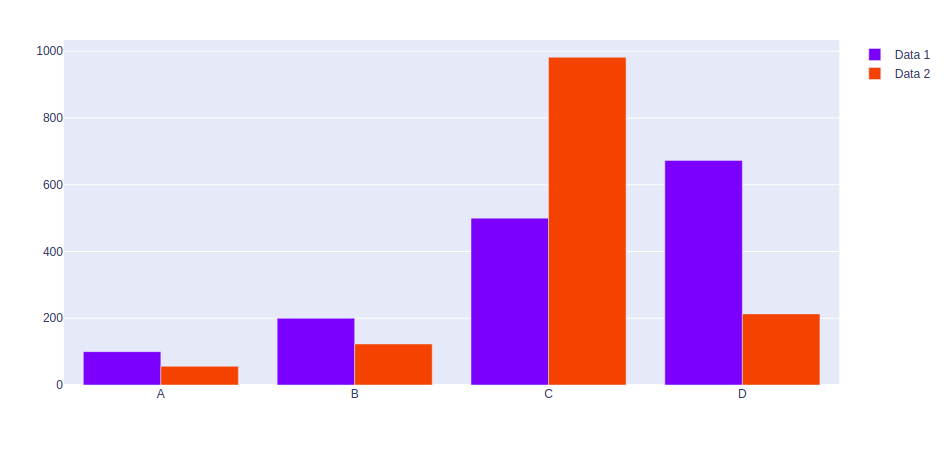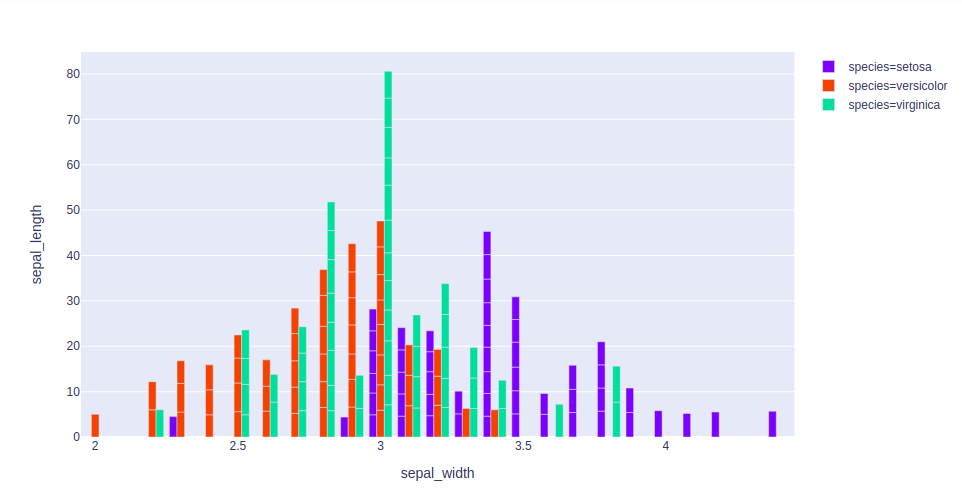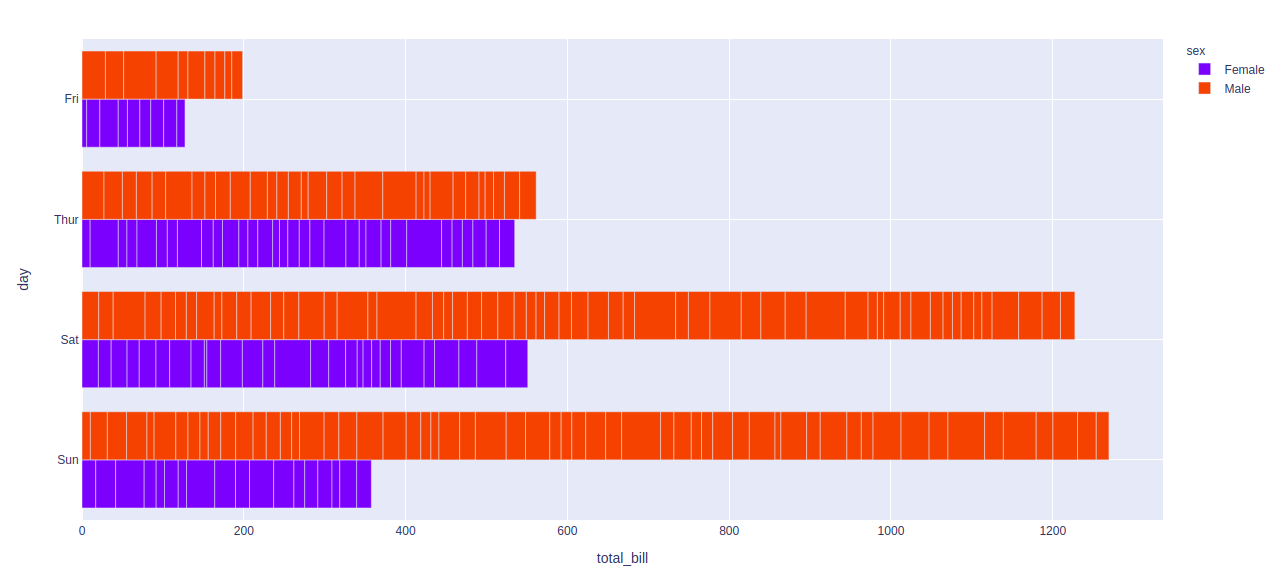# How to group Bar Charts in Python-Plotly?

Plotly is a Python library which is used to design graphs, especially interactive graphs. It can plot various graphs and charts like histogram, barplot, boxplot, spreadplot and many more. It is mainly used in data analysis as well as financial analysis. plotly is an interactive visualization library.

## Grouping Bar charts

Grouping bar charts can be used to show multiple set of data items which are been compared with a single color which is used to indicate a specific  series across all sets.

Method 1: Using graph_objects class

Example:

## Python3

 `import` `plotly.graph_objects as px` `import` `numpy`     `# creating random data through randomint ` `# function of numpy.random ` `np.random.seed(``42``)`   `random_x``=` `np.random.randint(``1``,``101``,``100``) ` `random_y``=` `np.random.randint(``1``,``101``,``100``)`   `x ``=` `[``'A'``, ``'B'``, ``'C'``, ``'D'``]`   `plot ``=` `px.Figure(data``=``[px.Bar(` `    ``name ``=` `'Data 1'``,` `    ``x ``=` `x,` `    ``y ``=` `[``100``, ``200``, ``500``, ``673``]` `   ``),` `                       ``px.Bar(` `    ``name ``=` `'Data 2'``,` `    ``x ``=` `x,` `    ``y ``=` `[``56``, ``123``, ``982``, ``213``]` `   ``)` `])` `                 `  `plot.show()`

Output:Method 2: Using express class

Example 1: Iris dataset

## Python3

 `import` `plotly.express as px` ` `  `df ``=` `px.data.iris()` ` `  `fig ``=` `px.bar(df, x``=``"sepal_width"``, y``=``"sepal_length"``,` `             ``color``=``"species"``, hover_data``=``[``'petal_width'``],` `             ``barmode ``=` `'group'``)` ` `  `fig.show()`

Output:Example 2: Tips dataset

## Python3

 `import` `plotly.express as px`   `df ``=` `px.data.tips()`   `fig ``=` `px.bar(df, x``=``"tota_bill"``, y``=``"day"``, ` `             ``color``=``"sex"``, barmode ``=` `'group'``)`   `fig.show()`

Output:Whether you're preparing for your first job interview or aiming to upskill in this ever-evolving tech landscape, GeeksforGeeks Courses are your key to success. We provide top-quality content at affordable prices, all geared towards accelerating your growth in a time-bound manner. Join the millions we've already empowered, and we're here to do the same for you. Don't miss out - check it out now!

Previous
Next